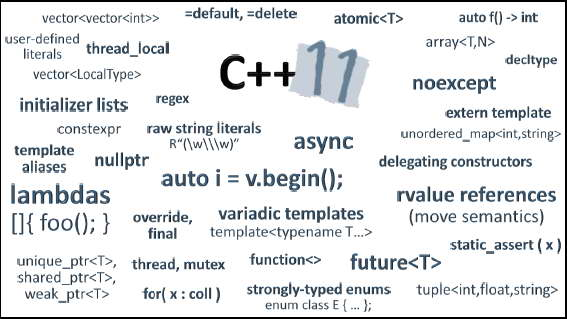# Khan's Notebook GCC/GNU/Linux Delphi/Window Java/Anywhere

## C++开发者都应该使用的10个C++11特性

【感谢冯上（@治不好你我就不是兽医 ）的热心翻译。如果其他朋友也有不错的原创或译文，可以尝试推荐给伯乐在线。】

### auto

 123 `auto i = 42;        ``// i is an int``auto l = 42LL;      ``// l is an long long``auto p = ``new` `foo(); ``// p is a foo*`

 1234 `std::map> map;``for``(auto it = begin(map); it != end(map); ++it) ``{``}`

 123456 `template` `<``typename` `T1, ``typename` `T2>``auto compose(T1 t1, T2 t2) -> decltype(t1 + t2)``{``   ``return` `t1+t2;``}``auto v = compose(2, 3.14); ``// v's type is double`

### nullptr

 123456789101112131415 `void` `foo(``int``* p) {}` `void` `bar(std::shared_ptr<``int``> p) {}` `int``* p1 = NULL;``int``* p2 = nullptr;   ``if``(p1 == p2)``{``}` `foo(nullptr);``bar(nullptr);` `bool` `f = nullptr;``int` `i = nullptr; ``// error: A native nullptr can only be converted to bool or, using reinterpret_cast, to an integral type`

### Range-based for loops （基于范围的for循环）

 12345678910111213141516171819202122 `std::map> map;``std::vector<``int``> v;``v.push_back(1);``v.push_back(2);``v.push_back(3);``map[``"one"``] = v;` `for``(``const` `auto& kvp : map) ``{``  ``std::cout << kvp.first << std::endl;` `  ``for``(auto v : kvp.second)``  ``{``     ``std::cout << v << std::endl;``  ``}``}` `int` `arr[] = {1,2,3,4,5};``for``(``int``& e : arr) ``{``  ``e = e*e;``}`### Override和final

 1234567891011 `class` `B ``{``public``:``   ``virtual` `void` `f(``short``) {std::cout << ``"B::f"` `<< std::endl;}``};` `class` `D : ``public` `B``{``public``:``   ``virtual` `void` `f(``int``) {std::cout << ``"D::f"` `<< std::endl;}``};`

D::f 按理应当重写 B::f。然而二者的声明是不同的，一个参数是short，另一个是int。因此D::f（原文为B::f，可能是作者笔误——译者注）只是拥有同样名字的另一个函数（重载）而不是重写。当你通过B类型的指针调用f()可能会期望打印出D::f，但实际上则会打出 B::f 。

 1234567891011 `class` `B ``{``public``:``   ``virtual` `void` `f(``int``) ``const` `{std::cout << ``"B::f "` `<< std::endl;}``};` `class` `D : ``public` `B``{``public``:``   ``virtual` `void` `f(``int``) {std::cout << ``"D::f"` `<< std::endl;}``};`

1. override，表示函数应当重写基类中的虚函数。
2. final，表示派生类不应当重写这个虚函数。

 1234567891011 `class` `B ``{``public``:``   ``virtual` `void` `f(``short``) {std::cout << ``"B::f"` `<< std::endl;}``};` `class` `D : ``public` `B``{``public``:``   ``virtual` `void` `f(``int``) override {std::cout << ``"D::f"` `<< std::endl;}``};`

 1 `'D::f' : method with override specifier 'override' did not override any base class methods`

 1234567891011121314151617 `class` `B ``{``public``:``   ``virtual` `void` `f(``int``) {std::cout << ``"B::f"` `<< std::endl;}``};` `class` `D : ``public` `B``{``public``:``   ``virtual` `void` `f(``int``) override final {std::cout << ``"D::f"` `<< std::endl;}``};` `class` `F : ``public` `D``{``public``:``   ``virtual` `void` `f(``int``) override {std::cout << ``"F::f"` `<< std::endl;}``};`

### Strongly-typed enums 强类型枚举

 12 `enum` `class` `Options {None, One, All};``Options o = Options::All;`

### Smart Pointers 智能指针

• unique_ptr: 如果内存资源的所有权不需要共享，就应当使用这个（它没有拷贝构造函数），但是它可以转让给另一个unique_ptr（存在move构造函数）。
• shared_ptr:  如果内存资源需要共享，那么使用这个（所以叫这个名字）。
• weak_ptr: 持有被shared_ptr所管理对象的引用，但是不会改变引用计数值。它被用来打破依赖循环（想象在一个tree结构中，父节点通过一个共享所有权的引用(chared_ptr)引用子节点，同时子节点又必须持有父节点的引用。如果这第二个引用也共享所有权，就会导致一个循环，最终两个节点内存都无法释放）。

 1234567891011121314 `void` `foo(``int``* p)``{``std::cout << *p << std::endl;``}``std::unique_ptr<``int``> p1(``new` `int``(42));``std::unique_ptr<``int``> p2 = std::move(p1); ``// transfer ownership` `if``(p1)``foo(p1.get());` `(*p2)++;` `if``(p2)``foo(p2.get());`

 123456789101112 `void` `foo(``int``* p)``{``}``void` `bar(std::shared_ptr<``int``> p)``{``++(*p);``}``std::shared_ptr<``int``> p1(``new` `int``(42));``std::shared_ptr<``int``> p2 = p1;` `bar(p1);``foo(p2.get());`

 1 `auto p3 = std::make_shared<``int``>(42);`

make_shared<T>是一个非成员函数，使用它的好处是可以一次性分配共享对象和智能指针自身的内存。而显示地使用shared_ptr构造函数来构造则至少需要两次内存分配。除了会产生额外的开销，还可能会导致内存泄漏。在下面这个例子中，如果seed()抛出一个错误就会产生内存泄漏。

 12345 `void` `foo(std::shared_ptr<``int``> p, ``int` `init)``{``*p = init;``}``foo(std::shared_ptr<``int``>(``new` `int``(42)), seed());`

 123456789101112 `auto p = std::make_shared<``int``>(42);``std::weak_ptr<``int``> wp = p;` `{``auto sp = wp.lock();``std::cout << *sp << std::endl;``}` `p.reset();` `if``(wp.expired())``std::cout << ``"expired"` `<< std::endl;`

### Lambdas

 1234567891011 `std::vector<``int``> v;``v.push_back(1);``v.push_back(2);``v.push_back(3);` `std::for_each(std::begin(v), std::end(v), [](``int` `n) {std::cout << n << std::endl;});` `auto is_odd = [](``int` `n) {``return` `n%2==1;};``auto pos = std::find_if(std::begin(v), std::end(v), is_odd);``if``(pos != std::end(v))``std::cout << *pos << std::endl;`

 1 `auto fib = [&fib](``int` `n) {``return` `n < 2 ? 1 : fib(n-1) + fib(n-2);};`
 1234 `error C3533: 'auto &': a parameter cannot have a type that contains 'auto'``error C3531: 'fib': a symbol whose type contains 'auto' must have an initializer``error C3536: 'fib': cannot be used before it is initialized``error C2064: term does not evaluate to a function taking 1 arguments`

 1 `std::function<``int``(``int``)> lfib = [&lfib](``int` `n) {``return` `n < 2 ? 1 : lfib(n-1) + lfib(n-2);};`

### 非成员begin()和end()

 123456789 `int` `arr[] = {1,2,3};``std::for_each(&arr, &arr+``sizeof``(arr)/``sizeof``(arr), [](``int` `n) {std::cout << n << std::endl;});` `auto is_odd = [](``int` `n) {``return` `n%2==1;};``auto begin = &arr;``auto end = &arr+``sizeof``(arr)/``sizeof``(arr);``auto pos = std::find_if(begin, end, is_odd);``if``(pos != end)``std::cout << *pos << std::endl;`

 1234567 `int` `arr[] = {1,2,3};``std::for_each(std::begin(arr), std::end(arr), [](``int` `n) {std::cout << n << std::endl;});` `auto is_odd = [](``int` `n) {``return` `n%2==1;};``auto pos = std::find_if(std::begin(arr), std::end(arr), is_odd);``if``(pos != std::end(arr))``std::cout << *pos << std::endl;`

 12345678910111213141516171819202122232425262728293031 `template` `<``typename` `Iterator>``void` `bar(Iterator begin, Iterator end)``{``std::for_each(begin, end, [](``int` `n) {std::cout << n << std::endl;});` `auto is_odd = [](``int` `n) {``return` `n%2==1;};``auto pos = std::find_if(begin, end, is_odd);``if``(pos != end)``std::cout << *pos << std::endl;``}` `template` `<``typename` `C>``void` `foo(C c)``{``bar(std::begin(c), std::end(c));``}` `template` `<``typename` `T, ``size_t` `N>``void` `foo(T(&arr)[N])``{``bar(std::begin(arr), std::end(arr));``}` `int` `arr[] = {1,2,3};``foo(arr);` `std::vector<``int``> v;``v.push_back(1);``v.push_back(2);``v.push_back(3);``foo(v);`

### static_assert和 type traits

static_assert提供一个编译时的断言检查。如果断言为真，什么也不会发生。如果断言为假，编译器会打印一个特殊的错误信息。

 12345678910111213 `template` `<``typename` `T, ``size_t` `Size>``class` `Vector``{``   ``static_assert(Size < 3, ``"Size is too small"``);``   ``T _points[Size];``};` `int` `main()``{``   ``Vector<``int``, 16> a1;``   ``Vector<``double``, 2> a2;``   ``return` `0;``}`